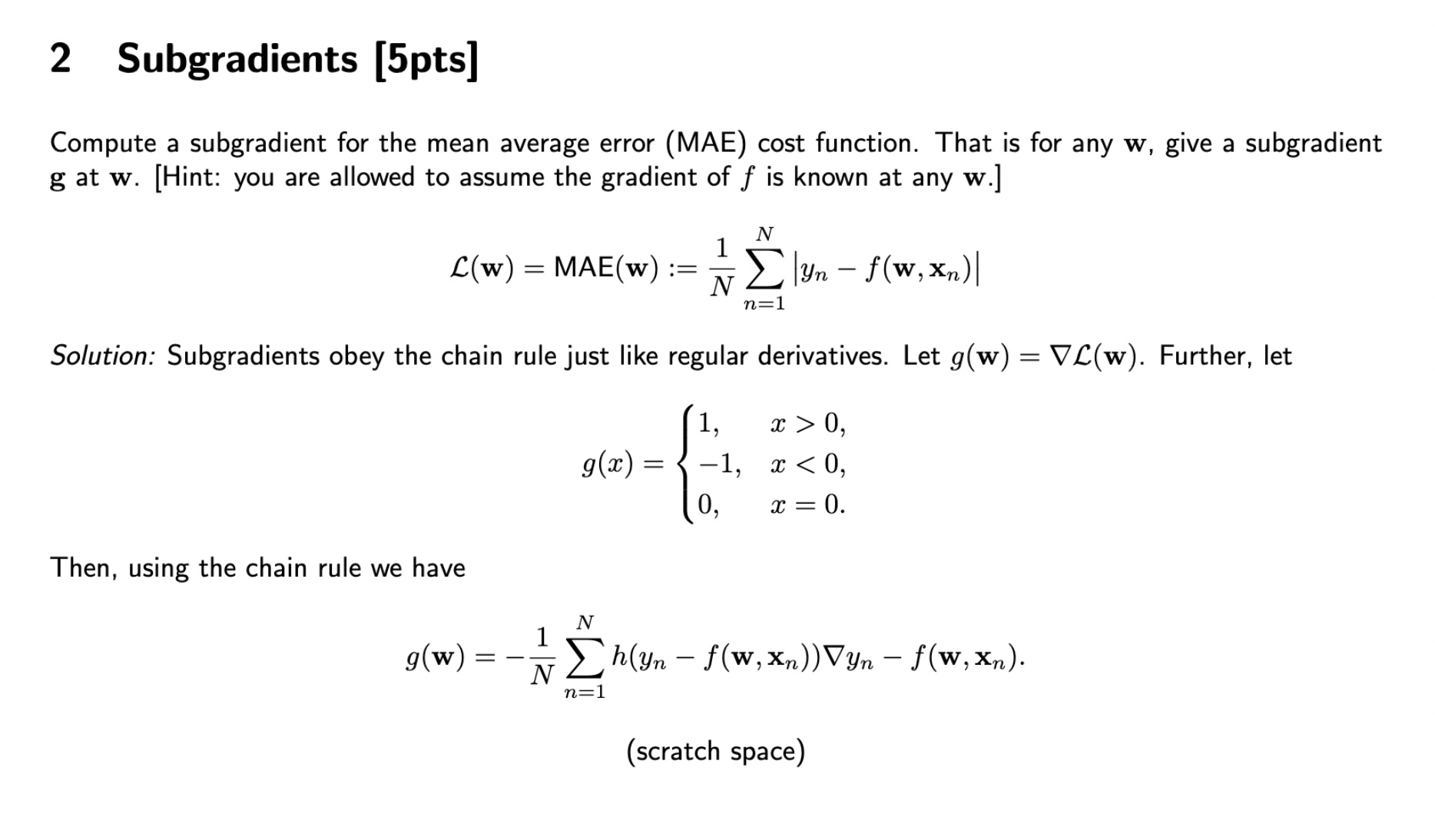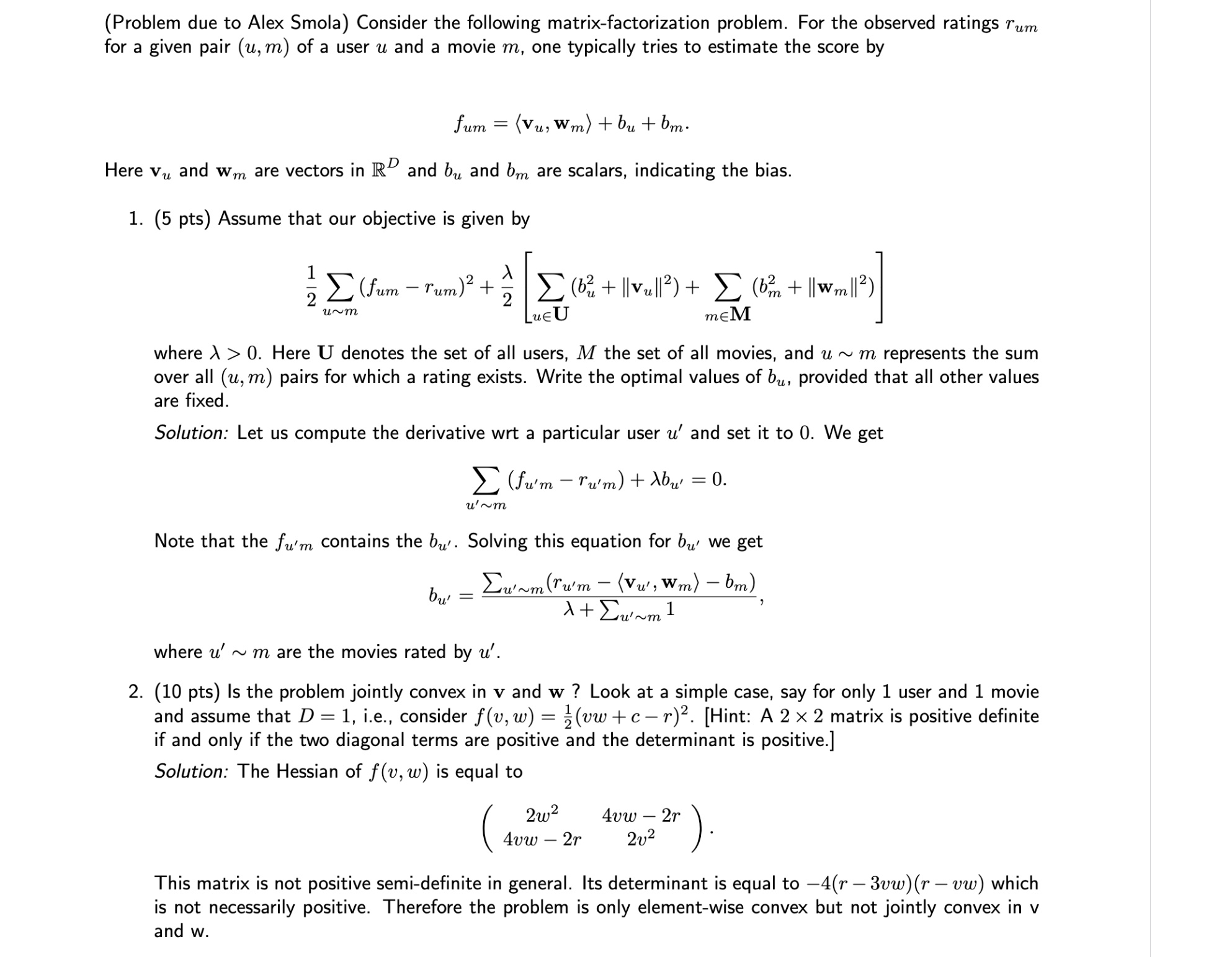### Open questions exam 2016

Hello,
For the subgradient problem, I am wondering if there is no a typo in the correction? In the final g(w), the h(.) corresponds to sign(.) right ? (so the g(x) defined above…?), and then maybe there is a parenthesis after the gradient for (y_n - f(w,xn))?
For the Matrix factorization problem, I do not see how they get their hessian, I do not have the same. And is this a generality that if the hessian is not PSD, the problem is element wise convex but not jointly convex in v and w? :/Top comment

Hi,

1. Yes, h corresponds to g, and yes there should be parenthesis after the gradient for (y_n - f(w,xn)).

2. If the hessian is not PSD, then we can only say that the problem is not convex over (v, w) jointly. We cannot imply anything about element-wise convexity. In this case the problem is element-wise convex because it is element-wise convex quadratic function.

3. Indeed, hessian is not correctly computed. To compute the hessian of f we first compute its partial derivatives:

$$\nabla_w f(v,w) = (vw + c - r)v; \nabla_v f(v,w) = (vw + c - r)w$$

Then second order derivatives:
$$\nabla^2_{w,w} f(w,w) = v^2; \nabla^2_{v,v} f(v,w) = w^2$$

$$\nabla^2_{w, v} f(v,w) = 2vw + c - r; \nabla^2_{v,w} f(v,w) = 2vw + c - r$$

Thus the hessian should be equal to:

(w^2 ; 2vw + c - r)
(2vw + c - r ; v^2)

Page 1 of 1